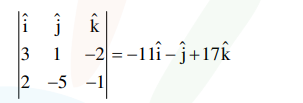# The equation of the plane passing through the

Question:

The equation of the plane passing through the point $(1,2,-3)$ and perpendicular to the planes $3 x+y-2 z=5$ and $2 x-5 y-z=7$, is

1. $3 x-10 y-2 z+11=0$

2. $6 x-5 y-2 z-2=0$

3. $11 x+y+17 z+38=0$

4. $6 x-5 y+2 z+10=0$

Correct Option: , 3

Solution:

Normal vector :So drs of normal to the required plane is

$<11,1,17>$

plane passes through $(1,2,-3)$

So eq $^{\mathrm{n}}$ of plane :

$11(x-1)+1(y-2)+17(z+3)=0$

$\Rightarrow \quad 11 x+y+17 z+38=0$